# Snap! thinks 18446744073709551615 = 18446744073709551614

Can anybody explain this?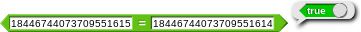Big numbers aren't normally stored accurately so computers can't tell the difference between them.

In Snap! - if you want to work with big numbers - load in the Big Integers library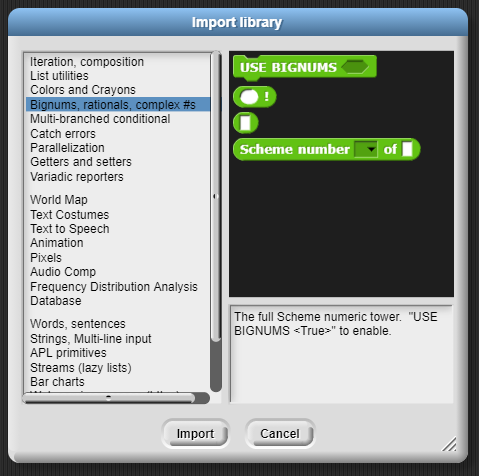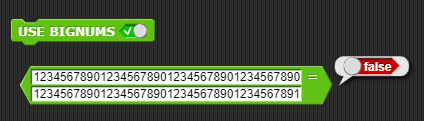@bh is right...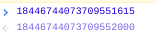18446744073709551615 returns a completely different number
But this: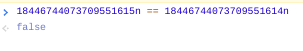But: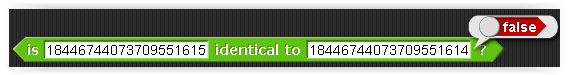you can use this instead of =

that's because identical checks if the two inputs are exactly the same, for example, 3 is not identical to 3.0. You should not use identical for math.

anyway, you don't use this for math:It's a true/false reporter...

If you want it for math you are using the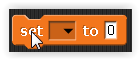block...

yeah... you're comparing two numbers. That's... that's not math. You're right.

What?

What?

Are you (@ego-lay_atman-bay) being sarcastic? If so, don't. Be nice. And remember that English is not @loucheman's native language.

But, @loucheman, what does using or not using SET have to do with whether or not something is mathematical? Also, "math" doesn't just mean arithmetic. When you say

I think you mean that when a block reports a Boolean value that makes it non-math. But true/false values are called "Boolean" after George Boole, the mathematician who gave logic its modern formal definition. The Boolean operators and the values False and True make an algebra in the same sense that a group or a field is an algebra.

Here is a true thing that maybe you meant: The = function is not an arithmetic operator in the formal meaning of that term, namely, a dyadic function whose range is the same as its domain. = is a function whose domain is (a superset of) numbers, but whose range is Booleans.

no. I wasn't really thinking clearly yesterday